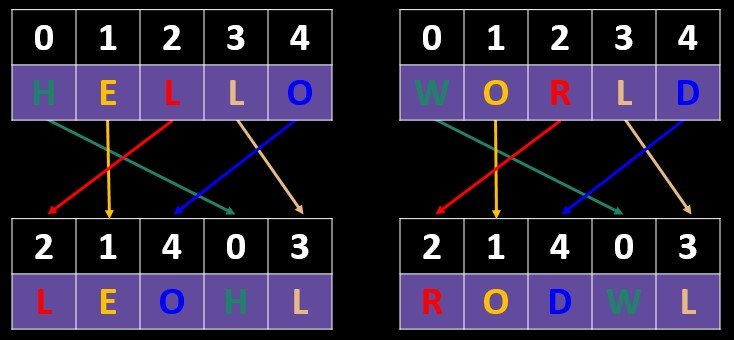# Transposition Cipher

Many ciphers obscure a plaintext by substituting the letters in the plaintext for alternative ones. A Caesar cipher, for example, might change ‘HELLO’ into ‘IFMMP’.

A transposition cipher does not substitute one letter for a different one, instead it obscures a plaintext by changing the position of each letter. The resulting ciphertext will contain all of the original letters from the plaintext, but in a different order.

There are many different ways to transpose a plaintext into a ciphertext.

## Simple transposition: anagram method

A simple transposition divides the plaintext into equal length blocks and then rearranges the letters within each block so the resulting letters are an anagram of the equivalent section from the original text. Each block is divided up in exactly the same way.The anagram is applied according to a key which refers to the positions of each letter. In the example above, which uses the key ‘2,1,4,0,3’, the first letter in the ciphertext is the letter that was at position 2 (i.e. the third letter) in the original text.

The key does not have to be 5 characters long – any length is possible. If the key is 5 characters long, then the number of possible anagrams that can be made is 5 x 4 x 3 x 2 x 1 = 120, i.e. 5!. This is because you have 5 choices for the first letter, then 4 remaining for the second letter and so on. The number of possible anagrams, being based on the factorial of the length of the key, grows very quickly:

• 5! = 120
• 6! = 720
• 7! = 5040
• 8! = 40320
• 9! = 362880
• 10! = 3628800
• 15! = 1307674368000
Tripling the key length from 5 to 15 characters, changes the number of possibilities from 120 to over 1.3 trillion!

### Cracking a simple transposition cipher

If the key used was quite short, say 10 digits or fewer, a brute force attack is possible. There are about 4 million different possibilities to check and this is not too difficult for a modern computer. The computer could apply each possible permutation to the ciphertext and then calculate a score for the transposed text, with the best score arising from the correctly transposed text. For more on how to calculate a score for cipher-cracking purposes, see here.

If the key is longer, then a brute force attack may no longer be feasible. In such cases, one approach for each key length could be to generate a random key for each key length, and then gradually change it to see if a higher score can be generated from the resulting transposed text. If the score decreases, reject the change and try another change; if it increases, keep the change and then try a new change to see if a further increase can be obtained. Occasionally, more drastic changes should be tried to stop the computer getting stuck on 'local maximums' - for more on this 'hill-climbing problem', see here.

There is, though, a neater way of cracking a transposition cipher with a longer key ...

Cipher Challenge competition    Leave feedback